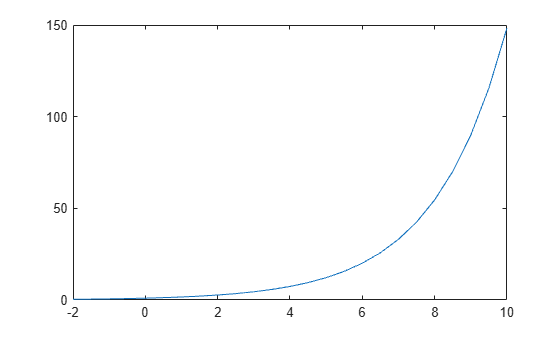exp

Description

example

Y = exp(X) returns the exponential ex for each element in array X. For complex elements z = x + iy, it returns the complex exponential

${e}^{z}={e}^{x}\left(\mathrm{cos}y+i\mathrm{sin}y\right)\text{\hspace{0.17em}}.$

Use expm to compute a matrix exponential.

Examples

collapse all

Calculate the exponential of 1, which is Euler's number, e.

exp(1)
ans = 2.7183

Euler's identity is the equality ${e}^{i\pi }+1=0$.

Compute the value of ${e}^{i\pi }$.

Y = exp(1i*pi)
Y = -1.0000 + 0.0000i

Plot $y={e}^{x/2}$ for x values in the range $\left[-2,10\right]$.

X = -2:0.5:10;
Y = exp(X/2);
plot(X,Y)Input Arguments

collapse all

Input array, specified as a scalar, vector, matrix, or multidimensional array.

Data Types: single | double
Complex Number Support: Yes

Output Arguments

collapse all

Exponential values, returned as a scalar, vector, matrix, or multidimensional array.

For real values of X in the interval (-Inf, Inf), Y is in the interval (0,Inf). For complex values of X, Y is complex. The data type of Y is the same as that of X.